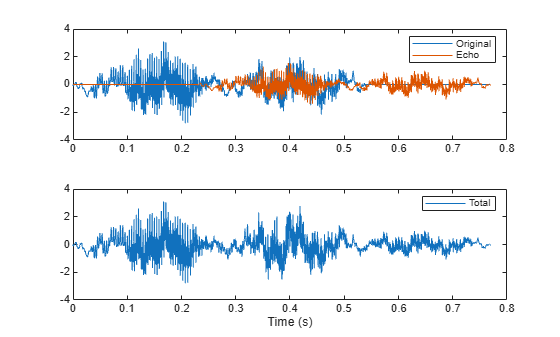Documentation

# rceps

Real cepstrum and minimum phase reconstruction

## Syntax

``[y,ym] = rceps(x)``
``rceps(x)``

## Description

example

````[y,ym] = rceps(x)` returns both the real cepstrum `y` and a minimum phase reconstructed version `ym` of the input sequence.```
````rceps(x)` returns the real cepstrum of the real sequence `x`.```

## Examples

collapse all

A speech recording includes an echo caused by reflection off a wall. Use the real cepstrum to filter it out.

In the recording, a person says the word MATLAB®. Load the data and the sample rate, ${F}_{s}=7418\phantom{\rule{0.2777777777777778em}{0ex}}Hz$.

```load mtlb % To hear, type soundsc(mtlb,Fs)```

Model the echo by adding to the recording a copy of the signal delayed by $\Delta$ samples and attenuated by a known factor $\alpha$: $y\left(n\right)=x\left(n\right)+\alpha x\left(n-\Delta \right)$. Specify a time lag of 0.23 s and an attenuation factor of 0.5.

```timelag = 0.23; delta = round(Fs*timelag); alpha = 0.5; orig = [mtlb;zeros(delta,1)]; echo = [zeros(delta,1);mtlb]*alpha; mtEcho = orig + echo;```

Plot the original, the echo, and the resulting signal.

```t = (0:length(mtEcho)-1)/Fs; subplot(2,1,1) plot(t,[orig echo]) legend('Original','Echo') subplot(2,1,2) plot(t,mtEcho) legend('Total') xlabel('Time (s)')````% To hear, type soundsc(mtEcho,Fs)`

Compute the real cepstrum of the signal. Plot the cepstrum and annotate its maxima. The cepstrum has a sharp peak at the time at which the echo starts to arrive.

```c = rceps(mtEcho); [px,locs] = findpeaks(c,'Threshold',0.2,'MinPeakDistance',0.2); clf plot(t,c,t(locs),px,'o') xlabel('Time (s)')```Cancel the echo by filtering the signal through an IIR system whose output, $w$, obeys $w\left(n\right)+\alpha w\left(n-\Delta \right)=y\left(n\right)$. Plot the filtered signal and compare it to the original.

```dl = locs(2)-1; mtNew = filter(1,[1 zeros(1,dl-1) alpha],mtEcho); subplot(2,1,1) plot(t,orig) legend('Original') subplot(2,1,2) plot(t,mtNew) legend('Filtered') xlabel('Time (s)')````% To hear, type soundsc(mtNew,Fs)`

## Input Arguments

collapse all

Input signal, specified as a real vector.

## Output Arguments

collapse all

Real cepstrum, returned as a vector.

Minimum phase real cepstrum, returned as a vector.

## Algorithms

The real cepstrum is the inverse Fourier transform of the real logarithm of the magnitude of the Fourier transform of a sequence.

### Note

`rceps` only works on real data.

`rceps` is an implementation of algorithm 7.2 in , that is,

```y = real(ifft(log(abs(fft(x))))); ```

Appropriate windowing in the cepstral domain forms the reconstructed minimum phase signal:

```w = [1;2*ones(n/2-1,1);ones(1-rem(n,2),1);zeros(n/2-1,1)]; ym = real(ifft(exp(fft(w.*y)))); ```

 Oppenheim, Alan V., and Ronald W. Schafer. Digital Signal Processing, Englewood Cliffs, NJ, Prentice-Hall, 1975.

 Programs for Digital Signal Processing, IEEE Press, New York, 1979.